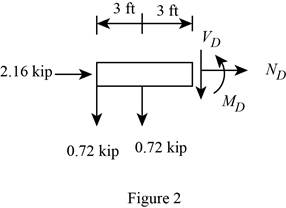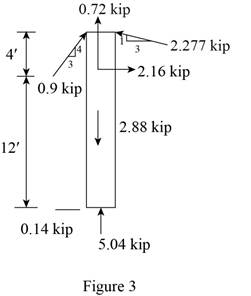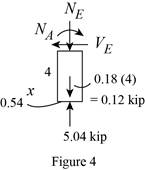Sample Solution from
Mechanics of Materials (10th Edition)
10th Edition
ISBN: 9780134319650
Chapter 1
Problem 1.1RP
Try another sample solutionarrow_forward
To determine

## To determine: The resultant internal loadings acting on cross sections located at D and E.

The resultant internal loadings at cross section at D are ND=2.16kip,VD=0_, and MD=2.16kipft_.

The resultant internal loadings at cross section at E are NE=4.32kip,VE=0.54kip_, and ME=2.16kipft_.

### Explanation of Solution

Explanation:

Given information:

The beam AB is pin supported at A and supported by a cable BC.

The weight of the beam AB is 120lb/ft.

The weight of the column FC is 180lb/ft.

Calculation:

PAB=Weight of beamAB×LengthofbeamAB

Substitute 120lb/ft for the weight of beam AB and 12 ft for the length of beam AB.

PAB=120×12=1,440lb

Convert the unit from lb to kip.

PAB=1,440lb×1kip1,000lb=1.44kip

Sketch the Free Body Diagram of the beam AB shown in Figure 1.Refer to Figure 1.

Find the angle of cable BC to the horizontal (θ):

sinθ=112+32sinθ=13.1623θ=sin1(0.3162)θ=18.43°

Find the tension in cable BC as shown below.

Take moment about A is Equal to zero.

MA=0(FBCsin18.43°×12)+1.44×6=03.794FBC+8.64=03.794FBC=8.64

FBC=2.277kip

Find the support reaction at A as shown below.

Apply the Equations of Equilibrium as shown below.

Summation of forces along horizontal direction is Equal to zero.

Fx=0Ax2.277cos18.43°=0Ax2.16=0Ax=2.16kip

Summation of forces along vertical direction is Equal to zero.

Fy=0Ay1.44+2.277sin18.43°=0Ay0.72=0Ay=0.72kip

Substitute 120lb/ft for the weight of beam AD and 6 ft for the length of beam AD.

Convert the unit from lb to kip.

Sketch the Free Body Diagram of the section for point D as shown in Figure 2.Refer to Figure 2.

Apply the Equations of Equilibrium as shown below.

Summation of forces along horizontal direction is Equal to zero.

Fx=0ND+2.16=0ND=2.16kip

Summation of forces along vertical direction is Equal to zero.

Fy=0VD+0.720.72=0VD=0

Take moment about D is Equal to zero.

MD=0MD0.72×3=0MD2.16=0MD=2.16kipft

Hence, the resultant internal loadings at cross section at D are ND=2.16kip,VD=0_, and MD=2.16kipft_.

PFC=Weight of columnFC×LengthofcolumnFC

Substitute 180lb/ft for the weight of column FC and 16 ft for the length of column FC.

PFC=180×16=2,880lb

Convert the unit from lb to kip.

PFC=2,880lb×1kip1,000lb=2.88kip

Sketch the Free Body Diagram of the beam FC shown in Figure 3.Refer to Figure 3.

Find the angle of cable CG to the horizontal.

sinθ=342+32sinθ=35θ=sin1(0.6)θ=36.87°

Find the tension in cable CG as shown below.

Summation of forces along horizontal direction is Equal to zero.

Fx=0FCGcos36.87°2.277sin18.43°=00.8FCG0.72=00.8FCG=0.72

FCG=0.9kip

PFE=Weight of columnFE×LengthofcolumnFE

Substitute 180lb/ft for the weight of column FE and 4 ft for the length of column FC.

PFE=180×4=720lb

Convert the unit from lb to kip.

PFE=720lb×1kip1,000lb=0.72kip

Sketch the Free Body Diagram of the section for point E as shown in Figure 4.Refer to Figure 4.

Apply the Equations of Equilibrium as shown below.

Summation of forces along horizontal direction is Equal to zero.

Fx=0VE0.54=0VE=0.54kip

Summation of forces along vertical direction is Equal to zero.

Fy=0NE+0.725.04=0NE4.32=0NE=4.32kip

Take moment about E is Equal to zero.

ME=0ME+0.54×4=0ME+2.16=0ME=2.16kipft

Therefore, the resultant internal loadings at cross section at E are NE=4.32kip,VE=0.54kip_, and ME=2.16kipft_.

Not sold yet?Try another sample solutionarrow_forward